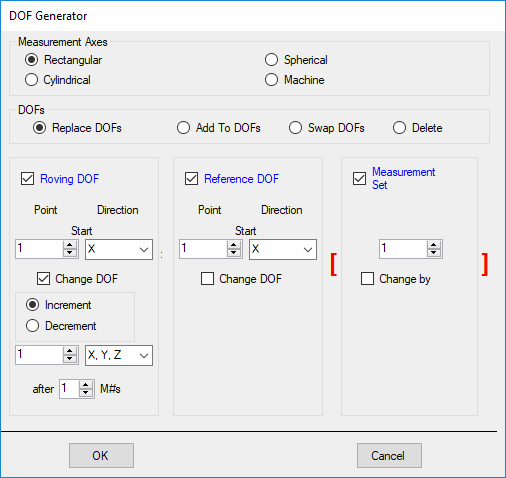# Using the DOF Generator

Used to create DOFs for all (or selected) M#s..

DOFs are optional.  They are used by certain MIMO commands, and for creating Animation equations by matching M# DOFs with Points & directions of a structure model.

• DOFs are displayed in the DOFs column of the M# spreadsheet.

• Double click on the DOFs column heading to open the DOF Generator dialog box.DOF Generator Dialog Box.

Each DOF has the format;  Roving DOF : Reference DOF [Measurement Set].

• For vibration or acoustic intensity data, a DOF consists of a Point number and a direction of measurement.

• For scalar data (temperature, pressure, voltage, current) a DOF consists only of a Point number.

• The Roving DOF precedes the colon ":" and the Reference DOF follows the colon ":".

• The Measurement Set number is enclosed in brackets [  ].

Measurement Set numbers are used to designate all measurements that were calculated from data that was simultaneously acquired.

Measurement directions depend on the type of Measurement Axes used. The notations shown in the Table below are used.

 Measurement Axes Direction Symbols Rectangular X, Y, Z Cylindrical R (radial), T (theta angle), Z (axial) Spherical R (radial), T (theta angle), P (phi angle) Machine H (horizontal), V (vertical), A (axial)

## Single Channel DOFs

Single channel measurements (e.g. Auto spectrum , PSD ) only require a Roving DOF.  To generate single channel DOFs using the DOF Generator,

• Choose Measurement Axes (Rectangular, Cylindrical, Spherical or Machine).

• Choose Replace With.

• Check Roving DOF, un-check Reference DOF, un-check Measurement Set.

• Enter a Point number & Direction in the Start boxes under Roving DOF.

• Check Increment under the Roving DOF.

• If the Data Type is Translation or Rotational, enter a Point number & direction in the Increment boxes under Roving DOF.

• If the Data Type is Scalar, enter a Point number in the Increment box under Roving DOF.

• If the DOF list doesn't contain the correct sequence of directions, type in the desired sequence.

• Enter the number of M# ’s after which the Roving DOF is to be incremented.  For example, if you enter 10, the same Roving DOF will be given to the first 10 M#’s.

• Click on OK to generate new DOFs.

## Cross Channel DOFs

Cross-channel measurements (e.g. FRF or Cross Spectrum) have both a Roving DOF and a Reference DOF.  To generate cross channel DOFs,

• Choose Measurement Axes (Rectangular, Cylindrical, Spherical or Machine).

• Choose Replace With.

• Check Roving DOF, check Reference DOF, un-check Measurement Set.

• Setup both the Roving DOF and Reference DOF sections as described above.

• Click on OK to generate new DOFs.

Retains a portion of each existing DOF, and either replaces or adds to the DOF.

### Roving Impact Test

If your measurements were acquired using a roving impact hammer,

• Each Roving DOF should be a hammer impact Point & direction.

• The Reference DOF should be the fixed accelerometer DOF.

## Swap DOFs

If your DOFs have a fixed Roving DOF and variable Reference DOFs, you will want to swap them.  The DOF preceding the colon is the Roving (changing) DOF and the DOF following the colon is the Reference (fixed) DOF

• Choose Swap in the DOF Generator, and click on OK.

## Delete DOFs

Deletes the DOFs of all (or selected) M#s.